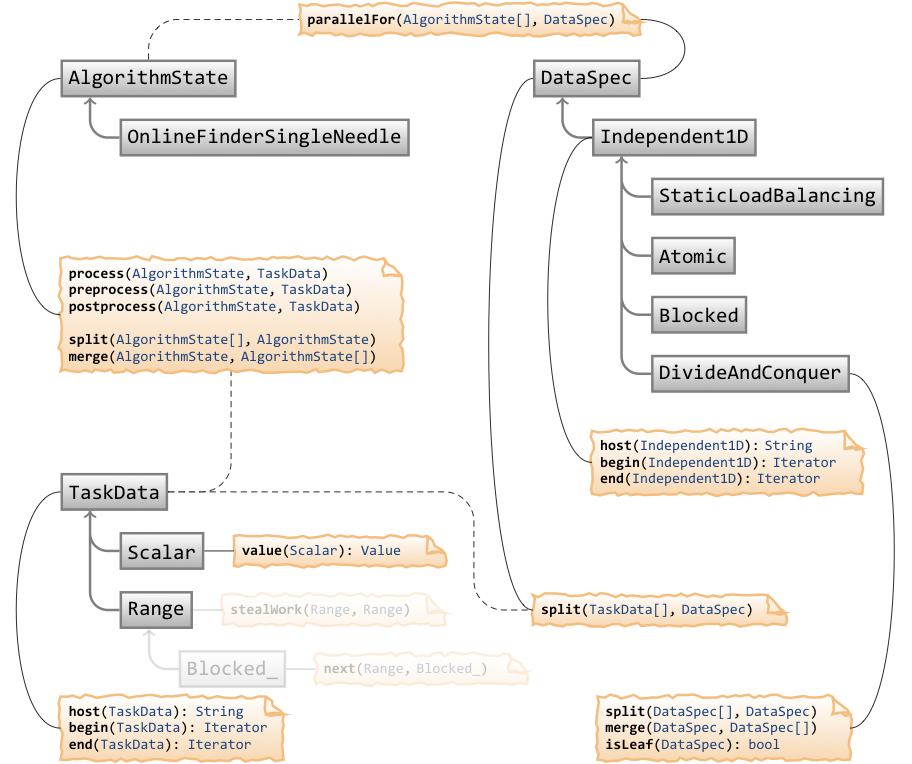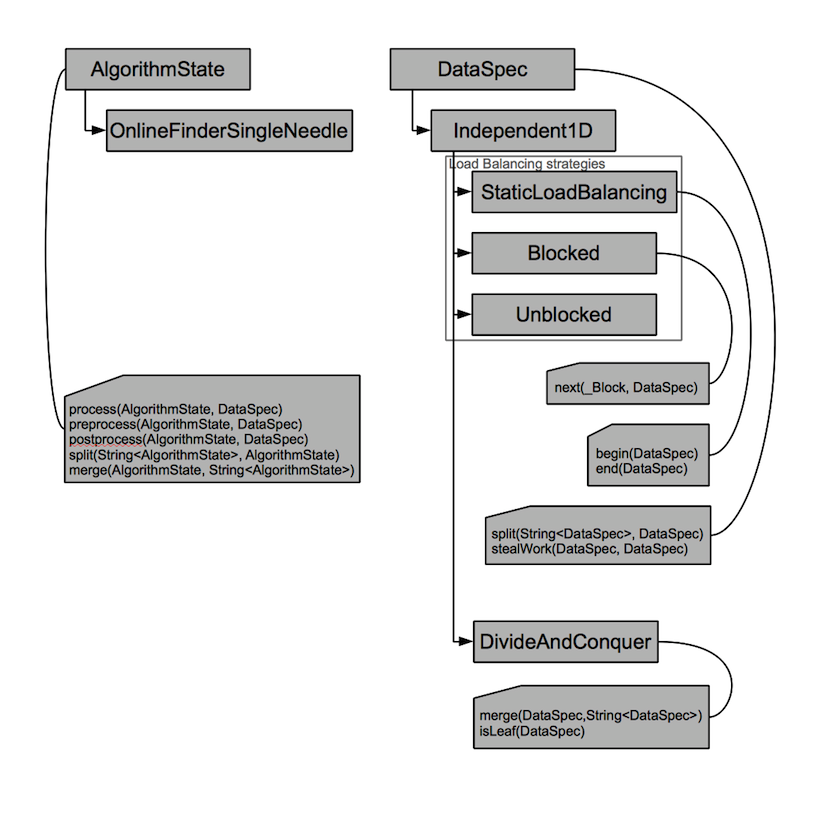# Open issues

• Providing N algorithm states to the `forall` method instead of just one introduces unnecessarily high cohesion. There are better ways of communicating the desired number of threads, if desired. Same goes for the output, which isn't merged yet.

Of course, this does make sense with fork/join parallelism but this breaks down for divide & conquer, or even earlier. Perhaps there should be a convenience wrapper around `forall` which takes just one algorithm state.

In any case, this will require a `split` and a `merge` method for algorithm states, as well.

• `split` ’s contract says that the size of the first argument is determined by the caller. Divide & conquer may be required to break that contract.

# New Version# Old version

## Interface Description## Data specification: `dataspec.h`

```// Common base class for parallel processing data specifications.
template <typename TSpec>
struct DataSpec;

// Split a data specification into several domains.
template <typename TSpec>
inline void
split(
// The receiving container of the split domains.
// The input size of this container determines the number of domains.
String<DataSpec<TSpec> >& specs,
// The data specification that is split into domains.
DataSpec<TSpec> const& spec
);

// One finshed task, identified by a data specification, attempts to steal work
// from another task that is still busy.
template <typename TSpec>
inline void
stealWork(
DataSpec<TSpec>& spec,
// The victim task that is stolen from.
DataSpec<TSpec>& other
);

// Data that has an independent 1D decomposition.
template <typename TString, typename TSpec>
struct Independent1D;

template <typename TString, typename TSpec>
struct DataSpec<Independent1D<TString, TSpec> > { };

template <typename TString, typename TSpec>
struct Iterator<DataSpec<Independent1D<TString, TSpec> >
: Iterator<TString> { };

template <typename TString, typename TSpec>
struct Iterator<DataSpec<Independent1D<TString, TSpec> const>
: Iterator<TString const> { };

template <typename TString, typename TSpec>
inline typename Iterator<DataSpec<Independent1D<TString, TSpec> > const>::Type
begin(DataSpec<Independent1D<TString, TSpec> > const& spec);

template <typename TString, typename TSpec>
inline typename Iterator<DataSpec<Independent1D<TString, TSpec> > >::Type
begin(DataSpec<Independent1D<TString, TSpec> >& spec);

template <typename TString, typename TSpec>
inline typename Iterator<DataSpec<Independent1D<TString, TSpec> > const>::Type
end(DataSpec<Independent1D<TString, TSpec> > const& spec);

template <typename TString, typename TSpec>
inline typename Iterator<DataSpec<Independent1D<TString, TSpec> > >::Type
end(DataSpec<Independent1D<TString, TSpec> >& spec);
```

## Blocked independent 1D data decomposition: `dataspec1d_blocked.h`

```//
// Blocked independent 1D vector decomposition.
//

// A single block inside a blocked 1D data spec.
struct TagBlock_;
typedef Tag<TagBlock_> BlockTag;

template <typename TString>
struct DataSpec<Independent1D<TString, BlockTag>
: DataSpec<Independent1D<TString, void>
{ };

template <typename TString>
struct Block {
typedef DataSpec<Independent1D<TString, BlockTag> > Type;
};

// Data with independent 1D decomposition that is decomposed into adjacent blocks.
template <typename TSpec>
struct Blocked;

template <typename TString, typename TSpec>
struct DataSpec<Independent1D<TString, Blocked<TSpec> > >
: DataSpec<Independent1D<TString, void> >
{ };

template <typename TString, typename TSpec>
inline void
split(
String<DataSpec<Independent1D<TString, Blocked<TSpec> > > >& specs,
DataSpec<Independent1D<TString, Blocked<TSpec> > > const& spec
);

template <typename TString, typename TSpec>
inline bool
next(
typename Block<DataSpec<Independent1D<TString, Blocked<TSpec> > > >::Type& block
DataSpec<Independent1D<TString, Blocked<TSpec> > >& spec,
);

template <typename TString, typename TSpec>
inline void
stealWork(
DataSpec<Independent1D<TString, Blocked<TSpec> > >& spec
DataSpec<Independent1D<TString, Blocked<TSpec> > >& other
);
// Perform an algorithm in parallel on a vector data structure of independent
// data.
template <typename TAlgSpec, typename TString, typename TBlockedSpec>
inline void
forall(
// String containing one algorithm description for each thread.
// The algorithm descriptions have to be identical.
String<AlgorithmState<TAlgSpec> >& states,
// The parallel data specification that describes the underlying data
// to perform the algorithm on.
DataSpec<Independent1D<TString, Blocked<TBlockedSpec> > > const& dataspec,
) {
typedef DataSpec<Independent1D<TString, Blocked<TDataSpec> > > TDataSpec;
typedef typename TDataSpec::TBlock TBlock;
typedef typename Iterator<TDataSpec>::Iterator TIterator;

String<TDataSpec> specs;
resize(specs, length(states));
split(specs, dataspec);

{
for(; ;) {
TIterator previous = TIterator();

TBlock block;
while (next(block, spec)) {

if (current != begin(block))
preprocess(alg, block);

process(alg, block);

// TODO(klmr) When to postprocess?
postprocess(alg, block);

previous = end(block);
}

[[other = randomly determine other thread]];
if (not worksteal(spec, other))
break;
};
}
}
```

## Algorithm state: `algstate.h`

```// Base class for the state of a parallel algorithm.
// Specializitions of this class specify the algorithm to be performed.
// These classes also store the state pertaining to the algorithm.
template <typename TSpec>
struct AlgorithmState;

// Perform the algorithm on one individual task, identified by a data
// specification.
template <typename TAlgSpec, typename TDataSpec>
inline void
process(
// The algorithm state.
AlgorithmState<TAlgSpec> const& state,
// The task to be processed.
DataSpec<TDataSpec> const& spec
);

// Perform preprocessing on an individual task, if such preprocessing is
// necessary because the algorithm state either isn't initialized yet
// or if its state is stale.
//
// Staleness means that the algorithm state has no logical connection to the
// was processed by this algorithm state.
template <typename TAlgSpec, typename TDataSpec>
inline void
preprocess(
// The algorithm state.
AlgorithmState<TAlgSpec> const& state,
// The task to be preprocessed.
DataSpec<TDataSpec> const& spec
);

// Perform postprocessing on an individual task, if necessary because the
// overall algorithm has finished or the next task to be processed by this
// algorithm state has no logical connection to the current task.
template <typename TAlgSpec, typename TDataSpec>
inline void
postprocess(
// The algorithm state.
AlgorithmState<TAlgSpec> const& state,
// The task to be postprocessed.
DataSpec<TDataSpec> const& spec
);
```
Topic attachments
I Attachment Action Size Date Who Commentpng object-model-old.png manage 251 K 16 Sep 2010 - 17:15 UnknownUserpng object-model.png manage 231 K 16 Sep 2010 - 17:15 UnknownUser
Topic revision: r9 - 16 Sep 2010, KonradRudolph